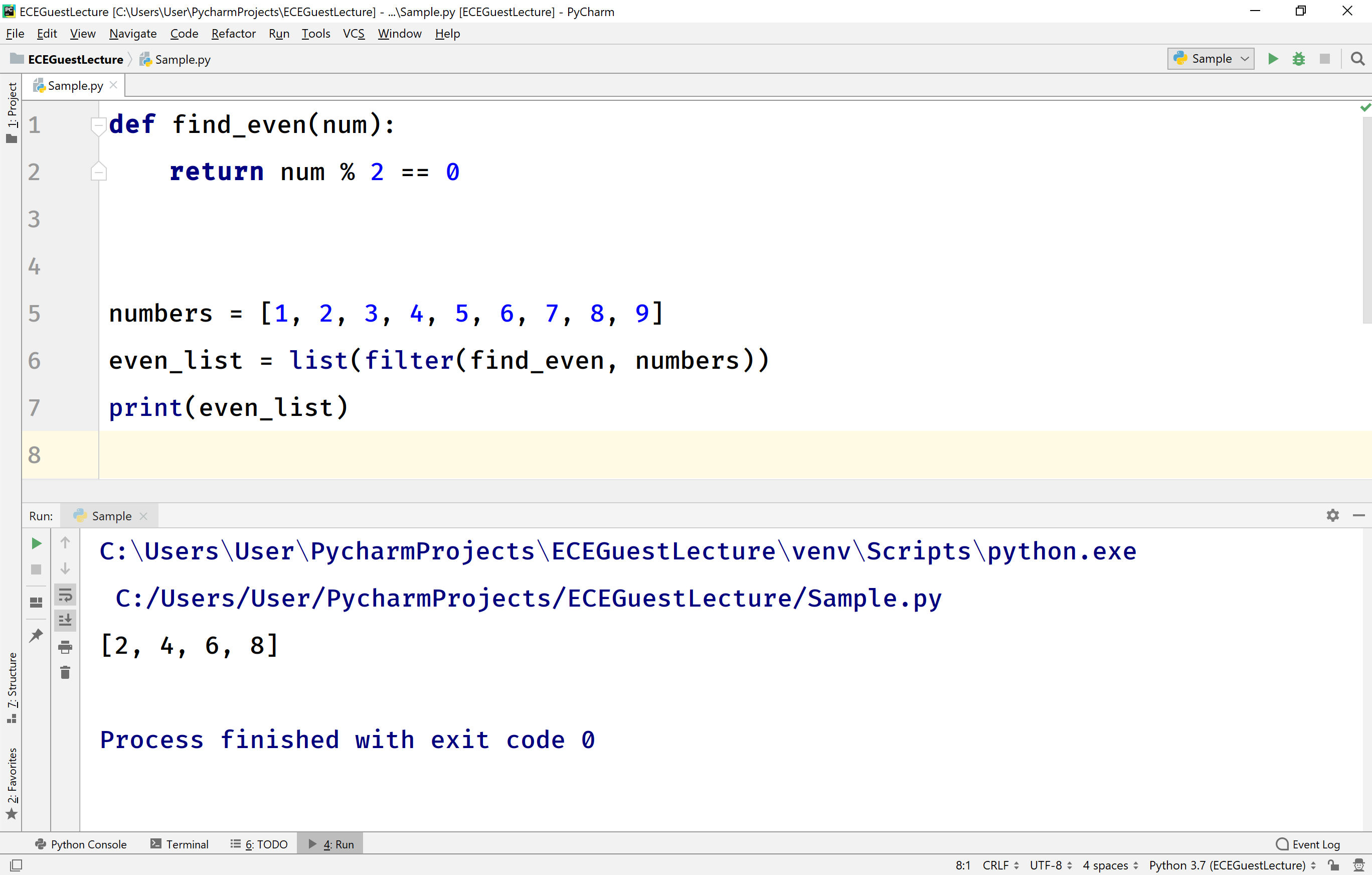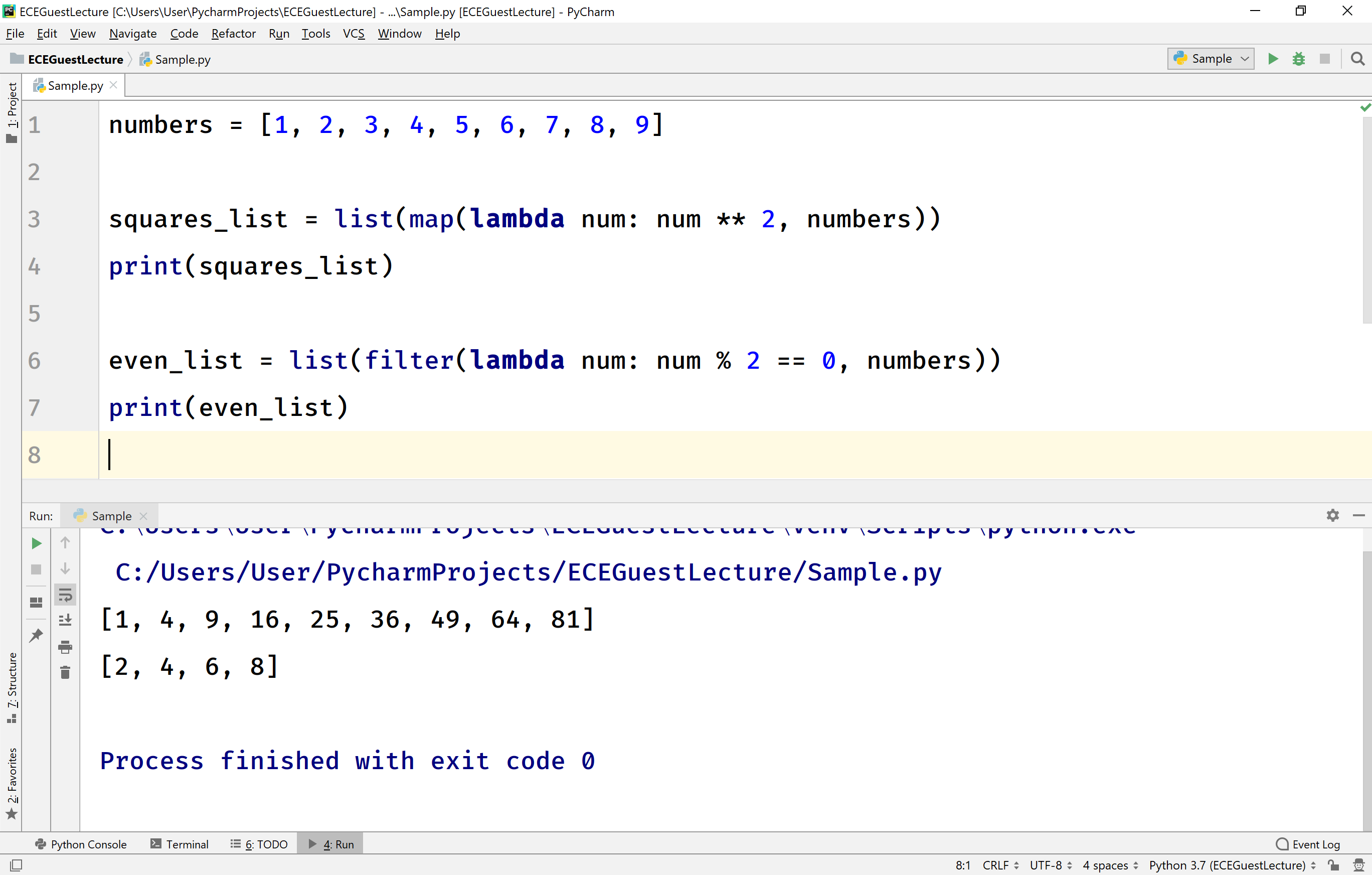The perfect place for easy learning...

×

# Python map and filter

In Python, the map( ) and filter( ) are the built-in functions used to execute a function for every value in a list. Both the built-in functions execute the specified function with the list element as an argument. Both map and filter functions return a list. Let us look at each function.

# map( ) function in Python

The general syntax to use map( ) function is as follows.

### Syntax

map(function_name, sequence_data_elements)

Here, map function accepts two arguments, the first argument is the function which is to be executed, and the second argument is the sequence of elements for which the specified functions have to be executed.

Points to be Remembered!
• The first argument must be only function name without any parenthesis.

Consider the following example code.

# Python code to illustrate map function.

``````def square(num):
return num ** 2

numbers = [1, 2, 3, 4, 5, 6, 7, 8, 9]
squares = list(map(square, numbers))
print(squares)```
```

In the above example code, the function square( ) is executed repeatedly passing every element from the numbers list. Finally, it returns the list of all return values.

When we run the above example code, it produces the following output.# filter( ) function in Python

The general syntax to use filter( ) function is as follows.

### Syntax

filter(function_name, sequence_data_elements)

Here, filter function accepts two arguments, the first argument is the function which is to be executed, and the second argument is the sequence of elements for which the specified functions have to be executed.

Points to be Remembered!
• The first argument must be a function which returns a boolean value only (either True or False).
• The first argument must be only function name without any parenthesis.
• The filter function returns a list consist of the arguments passed to the function for which it returns True.

Consider the following example code.

# Python code to illustrate filter function.

``````def find_even(num):
return num % 2 == 0

numbers = [1, 2, 3, 4, 5, 6, 7, 8, 9]
even_list = list(filter(find_even, numbers))
print(even_list)```
```

In the above example code, the function find_even( ) is executed repeatedly passing every element from the numbers list. Finally, it returns the list which contains all the arguments for which the function returns True.

When we run the above example code, it produces the following output.# lambda with map( ) and filter( ) functions

Most of the times the lambda expression is used with built-in functions map( ) and filter( ). Let us look at an example of lambda expresion used with built-in functions map( ) and filter( ).

# Python code to illustrate lambda with map and filter functions.

``````numbers = [1, 2, 3, 4, 5, 6, 7, 8, 9]

squares_list = list(map(lambda num: num ** 2, numbers))
print(squares_list)

even_list = list(filter(lambda num: num % 2 == 0, numbers))
print(even_list)```
```

When we run the above example code, it produces the following output.